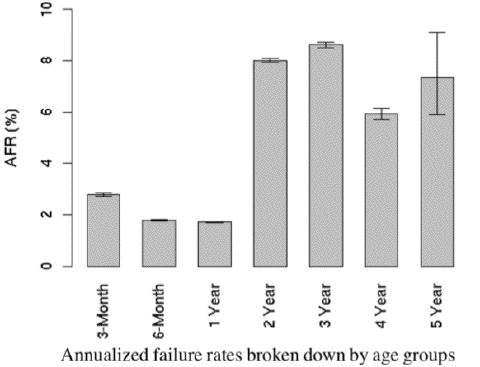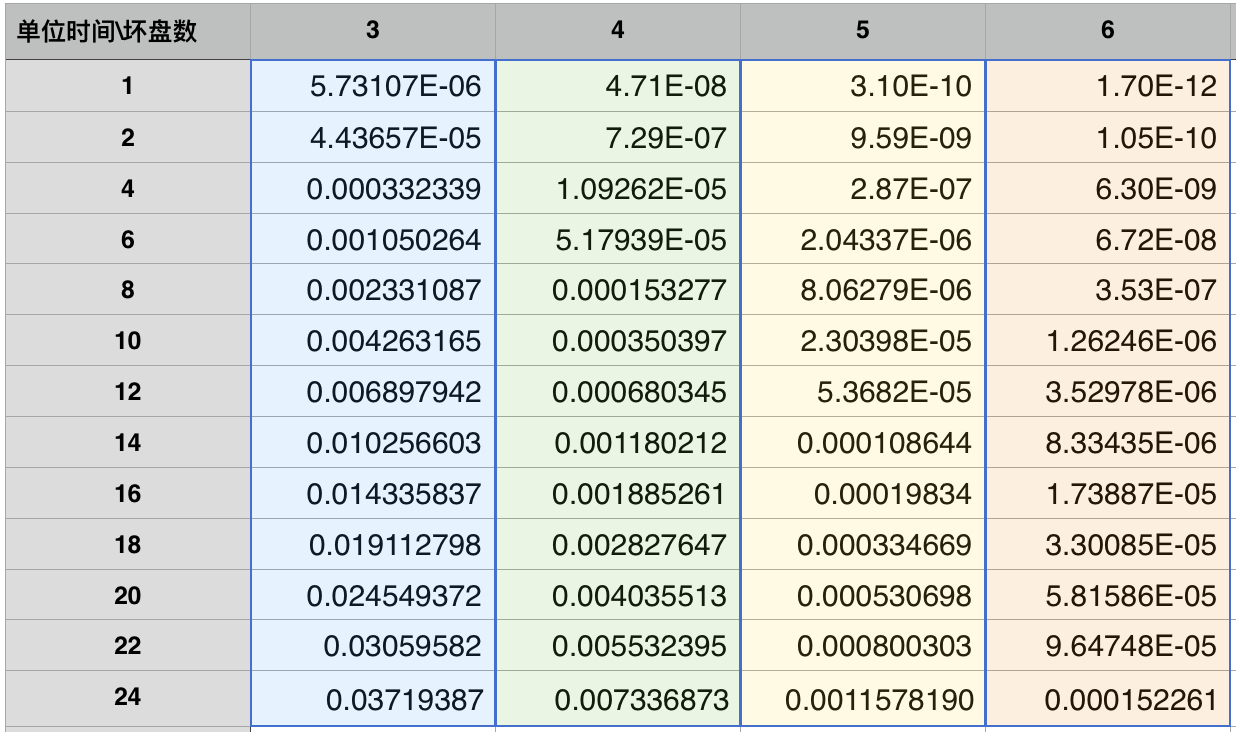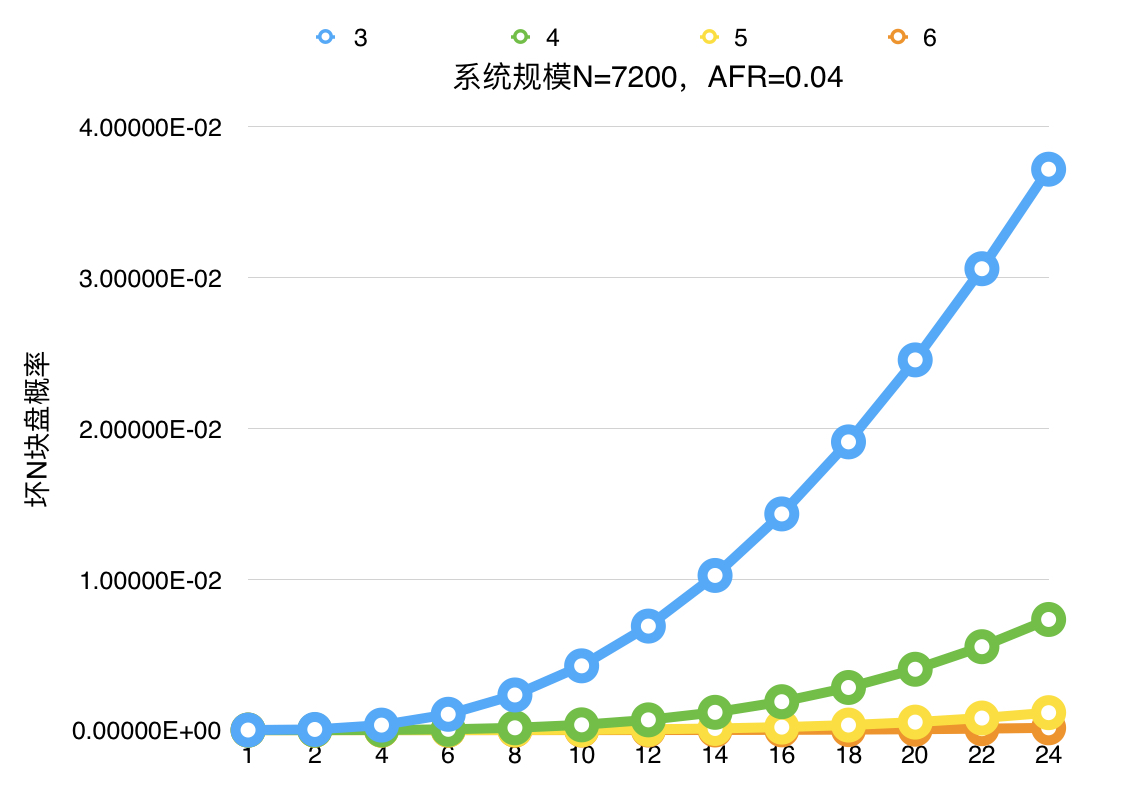2019/01/30

# 如何估算

## 数据丢失与copyset（复制组）

在999块磁盘3备份系统中，同时坏三块盘情况下的数据丢失概率?这个跟存储系统的设计息息相关。我们先考虑两个极端设计下的情况

CopySet：包含一个数据的所有副本数据的设备组合，比如一份数据写入1，2，3三块盘，那么{1,2,3}就是一个复制组。


9个磁盘的集群中，最小情况下的copyset的组合数为3，copysets = {1,2,3}、{4,5,6}、{7,8,9}，即一份数据的写入只能选择其中一个复制组，那么只有 {1,2,3}、{4,5,6}或者{7,8,9} 同时坏的情况下才会出现数据丢失。即最小copyset数量为N/R。

磁盘数量N，副本为R的存储系统中，copyset数量S, N/R < S  < C(N, R)


## 磁盘故障与存储系统可靠性估算

### 分布式存储系统中的丢失率估算

#### T时间内故障率

这里我们先定义一些数据

N: 磁盘数量
T: 统计时间
K: 坏盘数量
S: 系统中copyset数量(复制组的个数)
R: 备份数量


K个损坏情况下(随机选择K个盘情况下)命中复制组的概率为：

# 系统估算示例

## 估算示例

$S = Min(C_{7200}^{3}, \frac {7200 \times 8 \times 1024 \times 70\% }{3 \times 10 } ) = 1376256\\ Pa(T,K) = P(1,K) = \frac {X} {C_{7200}^K} \times P( N(1) = K)\\ \lambda = \frac { 4\% \times 7200} { 365 \times 24 } = 0.03\\ P(N(1) = K) = \frac {\lambda ^ K e ^ {-\lambda}} {K!}\\ Pb(T) = \sum _ {i=3} ^ {7200} {P(1,K)}\\ Pc = 1 - (1-Pb(T)) ^ {(365*24/1)}$

(按照4%的坏盘率计算7200块盘的系统中平均每小时的坏盘数量)

K P(N(1), K) P(1,K)
3 5.73e-6 1.26e-10
4 4.71e-8 ?
5 3.09e-10 ?
6 1.69e-12 ?
7 7.97e-15 ?
8 3.27e-17 ?
9 1.19e-19 ?

K P(N(1),K) P(1,K)
3 5.73e-6 1.26e-10
4 4.71e-8 4.17e-12
5 3.09e-10 6.85e-14

$Pb(T) \approx \sum _ {3}^{5}{P(1,K)} = 1.31 \times 10 ^ {-10}$ (即T=1小时内 丢数据的概率为1.31e-10)

$Pc = 1 - (1-Pb(T))^{\frac {365 \times 24}{T}} = 1.1 \times 10 ^{-06}$， 即6个9的可靠性。

#!/usr/bin/python
# -*- coding: utf-8 -*-
import decimal
import math
import time
# 随机分布情况下系统的copyset组合数
def RandomCopySets(N, DiskSize, RepNum, Percent, PartSize):
setNum = (N * DiskSize * Percent / (RepNum *  PartSize))
MaxCopySetNum = C(N, RepNum)
copysetNum = 0
if setNum > MaxCopySetNum:
copysetNum = MaxCopySetNum
else:
copysetNum =  setNum
return int(copysetNum)
# N 个磁盘存储系统中T时间同时损坏K块盘的概率,年故障率ARF
def  KdiskFailRate(N, T, ARF, K):
# λ 每小时的换盘数量
lambda1 = decimal.Decimal(str(N*AFR/24/365))
return poisson(lambda1, T, K)
# 副本数R的N 个磁盘存储系统中T时间内造成数据丢失的概率, 只统计R -> 2R-1个副本情况下的丢失数据概率(大于R个情况下，在一遍情况下对结果影响比较小)
def LossDataInT(S, N, RepNum, T, ARF):
loosRate = decimal.Decimal(str(0.0))
for k in range(RepNum, RepNum*2):
kdrate = KdiskFailRate(N,T,ARF,k)
singlerate = S * C(N-3, k-3)/C(N,k)
kdlossrate = kdrate * singlerate
print "k = " + str(k)  + ", " +str(kdrate) + ", " + str(kdlossrate)
loosRate += kdlossrate
print loosRate
return loosRate
# define loseRate in one Year
def LoseRate(S, N, RepNum, T, AFR):
return 1 - (1 - LossDataInT(S, N, RepNum, T, AFR))**(365*24/T)
#组合运算
def C(n, m):
return factorial(n) / (factorial(m)*factorial(n-m))
#泊松分布
def poisson(lam, t, R):
e=decimal.Decimal(str(math.e))
return ((lam * t) ** R) * (e**(-lam*t)) / factorial(R)
#t时间内损坏R块磁盘的概率
def probability(t, R):
return poisson(t, R)
#级数
def factorial(n):
S = decimal.Decimal("1")
for i in range(1, n+1):
N = decimal.Decimal(str(i))
S = S*N
return S
# case 1
N = 7200
DiskSize = 8*1024
Percent = 0.7
PartSize = 10
RepNum = 3
T = 1
AFR = 0.04
S  =  RandomCopySets(N, DiskSize, RepNum, Percent, PartSize)
print LoseRate(S, N, RepNum, T, AFR)


# 设计模式

LoseRate(S, N, RepNum, T, AFR)
N: 系统中磁盘的数量（包括磁盘的容量信息）
S: 系统Copyset的数量
RepNum: 存储的备份数量
T: 坏盘情况下的恢复时间
AFR: 磁盘的年度故障率


## 年故障率(AFR)1. 及时替换老的或者故障磁盘

在系统设计层面上需要能够记录每一块硬盘的品牌、系列、上线日期等，对于经常出现坏块和频繁出错的磁盘需要尽快进行，并且对于快要使用年限的磁盘进行替换下线。

2. 根据SMART信息预测换盘

采集磁盘的SMART的信息，分析SMART信息，对磁盘的换盘行为做预测工作。

RepNum 可靠性(年故障率)
3 1.14*10E-6
4 2.78*10E-8
5 3.18*10E-10
6 3.32*10E-12
7 2.33*10E-14

## Copyset数目(S)

CopySetsNum 可靠性(年故障率)
S 1.14*10E-6
S/2 5.74*10E-7
S/4 2.87*10E-7
S/8 1.43*10E-7
S/16 7.17*10E-8

## 修复时间(T)